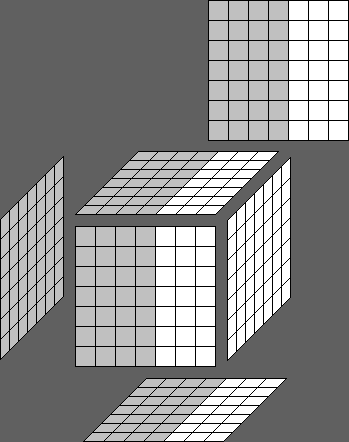HOME   DelTree | ExtractResources | Hash | IntegrityCheck | Jigoku Sudoku | Rays | Rubik's cube | SelectiveStartup About

# Rubik's cube solving

This page supplies the general instructions for using the solution guides on the website.

The images below show 7x7x7 cubes, but they hold for every cube. Larger cubes add more types
of moves.

### Commands

•  F = Front
•  B = Back
•  L = Left
•  R = Right
•  D = Down
•  U = Up
•  H = Horizontal
•  V = Vertical

### Elements

There are 4 elements involved in describing a move.

1.
A letter that tells you which side to rotate:
•  F = front side
•  U = upper side

2.
A superscript number or apostrophe tells you how many degrees to rotate and in which direction:
For F+B you have to imagine looking at the F side.
For L+R+V you have to imagine looking at the R side.
For U+D+H you have to imagine looking at the U side.
•  F   = front side 90 degrees clockwise
•  L'  = left side 90 degrees counterclockwise (as seen from the R side perspective)
•  H2 = single horizontal row 180 degrees
•  V'  = single vertical row 90 degrees counterclockwise (as seen from the R side perspective)

3.
A number that tells you which (single) layer to rotate:
•  F = 1st layer of the front side
•  2U = 2nd layer of the upper side
•  3L = 3rd layer of the left side

4.
Underlined tells you whether to rotate multiple layers simultaneously:
•  2F = 2 layers of the front side
•  3U = 3 layers of the upper side

Combined examples:
•  2F' = rotate 2 front side layers counterclockwise.
•  3L2 = rotate the 3rd left side 180 degrees.

### Basic moves

 F F' F2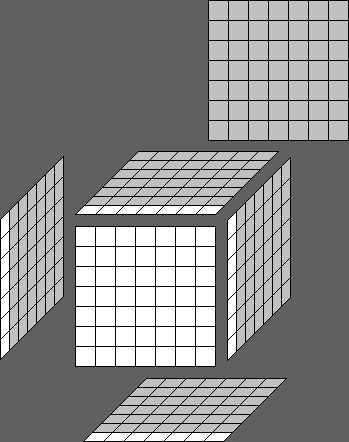B B' B2L L' L2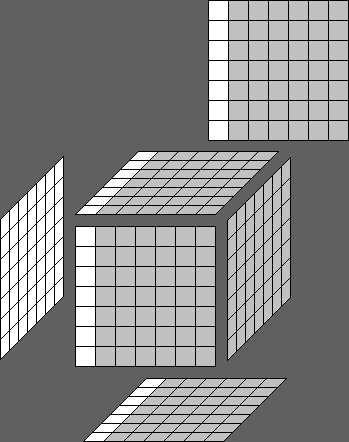R R' R2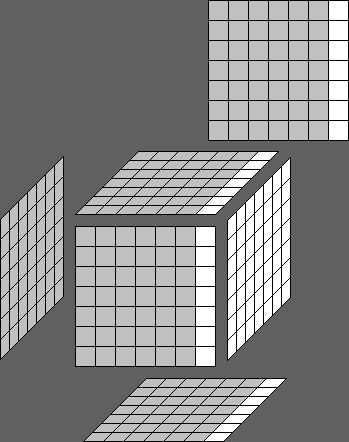D D' D2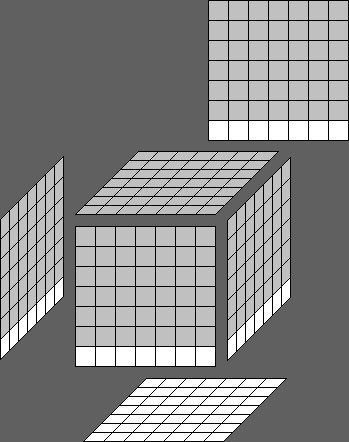U U' U2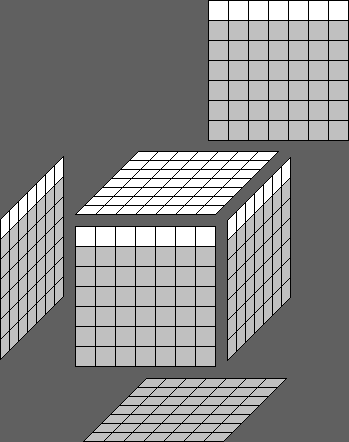H H' H2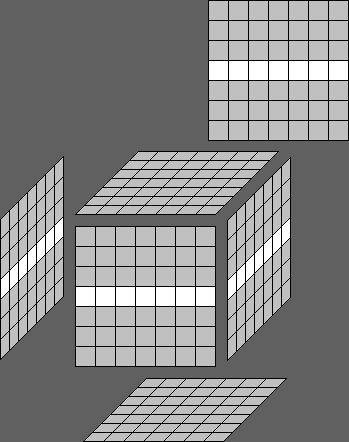V V' V2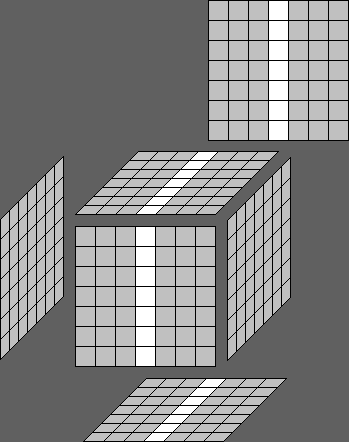2F 2F' 2F23R 3R' 3R2